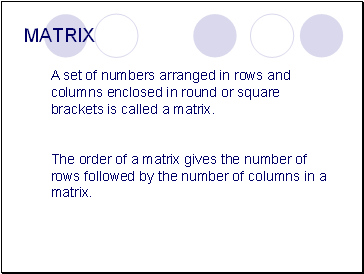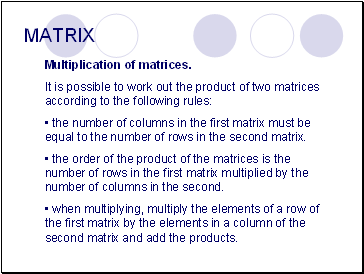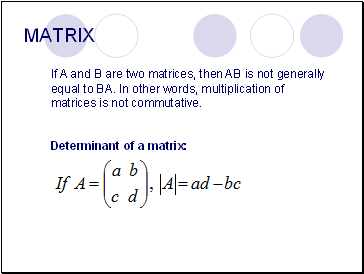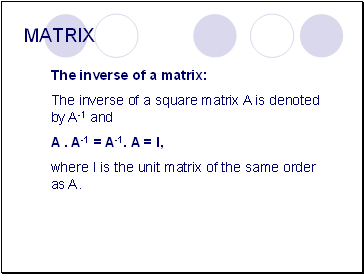# Matrix-transformationPage 1

#### WATCH ALL SLIDES

Slide 1## Matrix

A set of numbers arranged in rows and columns enclosed in round or square brackets is called a matrix.

The order of a matrix gives the number of rows followed by the number of columns in a matrix.

Slide 2MATRIX

A matrix with an equal number of rows and columns is called a square matrix.

A diagonal matrix has all its elements zero except for those in the leading diagonal (from top to bottom right).

Two matrices are equal if, and only if, they are identical. This means they must be of the same order and the respective elements must be identical.

Slide 3MATRIX

You can only add or subtract matrices of the same order.

To add, you simply add the corresponding elements in each matrix. To subtract, you subtract the corresponding elements in each matrix.

Scalar multiplication: You can multiply a matrix by a number. Each element of the matrix must be multiplied by the number.

Slide 4MATRIX

Multiplication of matrices.

It is possible to work out the product of two matrices according to the following rules:

the number of columns in the first matrix must be equal to the number of rows in the second matrix.

the order of the product of the matrices is the number of rows in the first matrix multiplied by the number of columns in the second.

when multiplying, multiply the elements of a row of the first matrix by the elements in a column of the second matrix and add the products.

Slide 5MATRIX

If A and B are two matrices, then AB is not generally equal to BA. In other words, multiplication of matrices is not commutative.

Determinant of a matrix:

Slide 6MATRIX

The inverse of a matrix:

The inverse of a square matrix A is denoted by A-1 and

A . A-1 = A-1. A = I,

where I is the unit matrix of the same order as A.